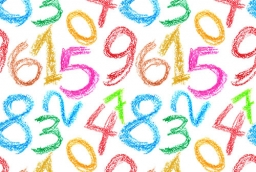# Determine 8611

Determine all natural numbers A and B pairs for which the sum of twice the least common multiple and three times the greatest common divisor of natural numbers A and B is equal to their product.

a1 =  5
b1 =  5
a2 =  9
b2 =  3

### Step-by-step explanation:Did you find an error or inaccuracy? Feel free to write us. Thank you!

Tips for related online calculators
Do you want to calculate the least common multiple of two or more numbers?
Do you want to calculate the greatest common divisor of two or more numbers?